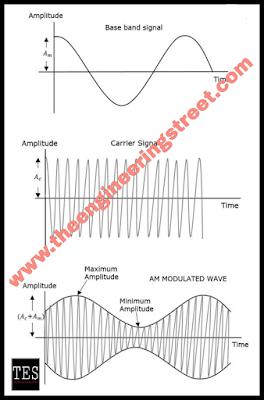## Friday, 5 July 2019

### What Is Amplitude Modulation and Benefits Of Amplitude Modulation With Their Advantages And Disadvantages And Also Different Types Of Amplitude Modulation

#### Amplitude Modulation(AM):

Before this topic we learn about modulation.....

what is modulation???

Modulation is a process the baseband signal is  superimpose on the carrier wave such that the frequency of baseband signal is same as that of carrier wave.(Baseband signal means message signal)

In order to long distance communication modulation process is used.

Benefits of modulation technique :
1. Reduction in the height of antenna.
2. Avoids mixing of signals.
3. Increases the range of communication.
4. Multiplexing is possible.
5. Improves quality of reception.
Types of modulation technique:
1. Amplitude modulation(AM)
2. Frequency modulation(FM)
3. Phase modulation(PM)

Here we learn about the amplitude modulation.

Topics we seen in this tutorial.....
• Introduction of AM
• Mathematical expression of AM
• Bandwidth of AM
• Application of AM

### Introduction of AM:

Modulation in which the amplitude of carrier signal is changes accordance to the amplitude of  the modulation signal.Amplitude modulation

In above figure first on shows the modulating wave, which is the message signal.

The next one is the carrier wave, which is a high frequency signal and contains no information.

While, the last one is the resultant modulated wave.i.e, amplitude modulated wave.

It can be observed that the positive and negative peaks of the carrier wave, are interconnected with an imaginary line.

### Mathematical expression of AM:

Let the modulating signal be,
and the carrier signal be,
c(t)=Acos(2πfct)
Where,
Am  and Ac are the amplitude of the modulating signal and the carrier signal .

fm and fc are the frequency of the modulating signal and the carrier signal respectively.

Then, the equation of Amplitude Modulated wave will be

#### s(t)=[Ac+Am cos⁡(2πfmt)]cos⁡(2πfct) (Eq. 1)

The ratio of amplitude of modulating signal and amplitude of carrier wave is called modulation index(M). It is also called as modulation depth.

From equation 1

s(t)=Ac[1+(Am/Ac)cos(2πfmt)]cos(2πfct)

s(t)=Ac[1+Mcos(2πfmt)]cos(2πfct)   (Eq. 2)

Where, M is Modulation index and it is equal to the ratio of Am and Ac(see earlier). Mathematically, we can write it as

#### M=Am/Ac (eq3)

Hence, we can calculate the value of modulation index by using the above formula.

Now, let see one more formula for Modulation index by considering Equation 1. We can use this formula for calculating modulation index value, when the maximum and minimum amplitudes of the modulated wave are known.

Let Amax and Amin be the maximum and minimum amplitudes of the modulated wave.
We will get the maximum amplitude of the modulated wave, when cos(2πfmt) is 1.

Amax = Ac+Am (Eq. 4)

We will get the minimum amplitude of the modulated wave, when cos(2πfmt) is -1.

Amin = Ac−Am (Eq. 5)

Add Equation 4 and Equation 5.

Amax+Amin = Ac+Am+Ac−Am = 2Ac

Ac=Amax+Amin/2  (Eq. 6)

Subtract Equation 5 from Equation 4.

Amax−Amin=Ac+Am−(Ac−Am) = 2Am

Am=Amax−Amin/2

The ratio of Equation 7 and Equation 6 will be as follows.

Am/Ac = [(Amax−Amin)/2] / [(Amax+Amin)/2]

M=Amax−Amin /Amax+Amin  (Eq. 8)

The modulation index  is often denoted in percentage called as Percentage of Modulation.

For a perfect modulation, the value of modulation index should be 1, which implies the percentage of modulation should be 100%.

### Bandwidth (BW):

It is the difference between the highest and lowest frequencies of the signal.

Mathematically, we can write it as

BW = fmax−fmin

Consider the following equation of amplitude modulated wave.

The amplitude modulated wave has three frequencies. Those are carrier frequency fc,
upper sideband frequency fc+fm and lower sideband frequency fc−fm
Here,
fmax = fc+fm and fmin = fc−fm

Substitute, fmax and fmin values in bandwidth formula.
BW = fc+fm−(fc−fm)

BW = 2fm

### Application of AM:

• It is widely used in broadcast transmission.
• The used of AM in aerospace in widespread.
• Hence, it is most of used in air band radio.

• It can be demodulated with less components.
• AM is easy to implement,i.e constuction is very easy.